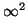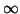Next: Nonlinear Factor Analysis Up: No Title Previous: Introduction

# Choosing Among Competing Explanations

Each model with particular values for sources, parameters and noise terms can be considered as an explanation for the observations. Even with linear PCA and ICA there are infinitely many possible models which explain the observations completely. With flexible nonlinear models like an MLP network, the number of possible explanations is -- loosely speaking -- even higher (although mathematically speaking,would still be `only').An example of competing explanations is given in Fig. 2. The data is sampled from an even distribution inside a square. This is equivalent to saying that two independent sources, each evenly distributed, have generated the data as shown on the left hand side of the figure. If we only look at the probability of the data, the nonlinear mapping depicted on the right hand side of the figure is even better explanation as it gives very high probabilities to exactly those data points that actually occurred. However, it seems intuitively clear that the nonlinear model in Fig. 2 is much more complex than the available data would justify.

The exact Bayesian solution is that instead of choosing a single model, all models are used by weighting them according to their posterior probabilities. In other words, each model is taken into account in proportion with how probable they seem in light of the observations.

If we look at the predictions the above two models give about future data points, we notice that the more simple linear model with two sources predicts new points inside the square but the more complex nonlinear model with one source predicts new points only along the curved line. The prediction given by the more simple model is evidently closer to the prediction obtained by the exact Bayesian approach where the predictions of all models would be taken into account by weighting them according to the posterior probabilities of the models.

With complex nonlinear models like MLP networks, the exact Bayesian treatment is computationally intractable and we are going to resort to ensemble learning, which is discussed in Chap. 6. In ensemble learning, a computationally tractable parametric approximation is fitted to the posterior probabilities of the models.

In Sect. 6.4.2 it is shown that ensemble learning can be interpreted as finding the most simple explanation for the observations. This agrees with the intuition that in Fig. 2, the simple linear model is better than the complex nonlinear model.

The fact that we are interested in simple explanations also explains why nonlinear ICA is needed at all if we can use nonlinear PCA. The nonlinearity of the mapping allows the PCA model to represent any time-independent probability density of the observations as originating from independent sources with Gaussian distributions. It would therefore seem that the non-Gaussian source models used in the nonlinear ICA cannot further increase the representational power of the model. However, for many naturally occurring processes the representation with Gaussian sources requires more complex nonlinear mappings than the representation with mixtures-of-Gaussians. Therefore the nonlinear ICA will often find a better explanation for the observations than the nonlinear PCA.

Similar considerations also explain why to use the MLP network for modelling the nonlinearity. Experience has shown that with MLP networks it is easy to model fairly accurately many naturally occurring multidimensional processes. In many cases the MLP networks give a more simple parametrisation for the nonlinearity than, for example, Taylor or Fourier series expansions.

On the other hand, it is equally clear that the ordinary MLP networks with sigmoidal nonlinearities are not the best models for all kinds of data. With the ordinary MLP networks it is, for instance, difficult to model mappings which have products of the sources. The purpose of this chapter is not to give the ultimate model for any data but rather to give a good model for many data, from which one can start building more sophisticated models by incorporating domain-specific knowledge. Most notably, the source models described here do not assume time-dependencies, which are often significant.Next: Nonlinear Factor Analysis Up: No Title Previous: Introduction
Harri Lappalainen
2000-03-03